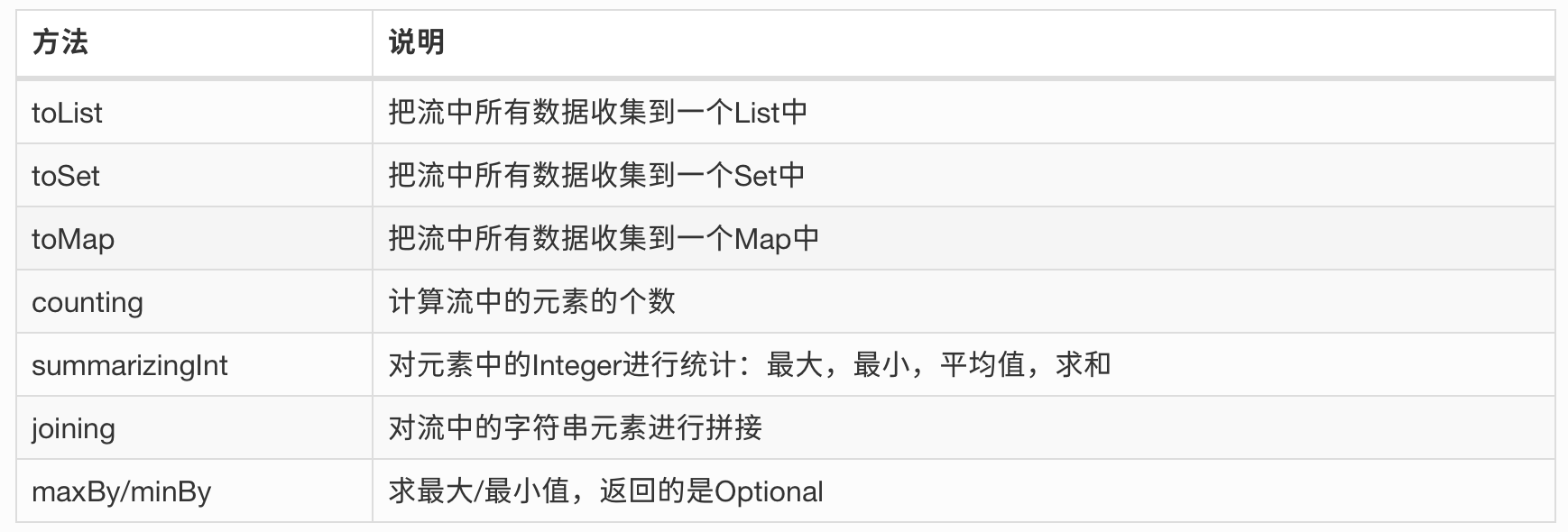# 可能是 Java Stream 的最佳实践（二）ES_her0

### 收集器

<R, A> R collect(Collector<? super T, A, R> collector);<R> R collect(Supplier<R> supplier,                  BiConsumer<R, ? super T> accumulator,                  BiConsumer<R, R> combiner);

### 收集器的超能力

• 汇总成一个值

• 分组

• 分区

#### reducing

List<User> users = Arrays.asList(        new User("John", 30),        new User("Julie", 35),        new User("Jack", 28));int totalAge1 = users.stream().collect(reducing(0,User::getAge,Integer::sum));int totalAge2 = users.stream().map(User::getAge).reduce(0, Integer::sum);int totalAge3 = users.stream().collect(summingInt(User::getAge));int totalAge4 = users.stream().mapToInt(User::getAge).sum();

String name1 = users.stream().collect(reducing("", User::getName, (s1, s2) -> s1 + s2));String name2 = users.stream().map(User::getName).collect(joining());#### groupingBy

//1Collector groupingBy(Function classifier);//2Collector groupingBy(Function classifier, Collector downstream);//3Collector groupingBy(Function classifier, Supplier mapFactory, Collector downstream);

groupingBy 一般需要配合 collect 使用，collect 方法如上文所述，接受一个 Collector 接口作为参数，这个接口我们稍后再说，现在只需要知道它也是个容器，用来装归约后的对象。

1. 直接传入 function，默认返回 Collector<T, ?, Map<K, List<T>>>，重点在于 Map<K, List<T>>，即组装成 Key-List 的格式

2. 第二种入参多了一个 Collector，可以做套娃：算总数，继续分组都可以。返回 Collector<T, ?, Map<K, D>>，第一种的 List 变成了一个 D，就是归约成了单个值，最后组装成了 K-V

3. 和第二种类似，但多了一个 Supplier，表示指定一个容器来存放归约后的值，返回值和第二种也一样

//准备数据public class User {  private String name;  private int age;  private String city;}List<User> users = Arrays.asList(        new User("John", 30, "Shanghai"),        new User("Julie", 30, "Beijing"),        new User("Jack", 28, "Beijing"),        new User("Jimmy", 28, "Beijing"),        new User("Mike", 20, "Nanjing"));

//第一种Map<Integer, List<User>> collect = users.stream().collect(Collectors.groupingBy(User::getAge));{  20=[{name = Mike, age = 20, city =Nanjing}],   28=[{name = Jack, age = 28, city =Beijing}, {name = Jimmy, age = 28, city =Beijing}],   30=[{name = John, age = 30, city =Shanghai}, {name = Julie, age = 30, city =Beijing}]}

//这里返回值有变化Map<Integer, Long> collect =  users.stream().collect(Collectors.groupingBy(User::getAge, Collectors.counting()));  {20=1, 28=2, 30=2}

Map<Integer, List<String>> collect = users.stream() .collect(Collectors.groupingBy(User::getAge,                                Collectors.mapping(User::getName,Collectors.toList()))); {  20=[Mike],   28=[Jack, Jimmy],   30=[John, Julie]}

Map<String, List<User>> collect =   users.stream()  .collect(Collectors.groupingBy(User::getCity, TreeMap::new, Collectors.toList()));{  Beijing=[{name = Julie, age = 30, city =Beijing}, {name = Jack, age = 28, city =Beijing}, {name = Jimmy, age = 28, city =Beijing}],   Nanjing=[{name = Mike, age = 20, city =Nanjing}],   Shanghai=[{name = John, age = 30, city =Shanghai}]}

Map<String, Map<Integer, List<User>>> collect = users.stream().collect(  Collectors.groupingBy(User::getCity,Collectors.groupingBy(User::getAge)));{Beijing=	{		28=[{name = Jack, age = 28, city =Beijing}, {name = Jimmy, age = 28, city =Beijing}], 		30=[{name = Julie, age = 30, city =Beijing}]	}, Shanghai=	{		30=[{name = John, age = 30, city =Shanghai}]	}, Nanjing=	{		20=[{name = Mike, age = 20, city =Nanjing}]	}}

#### partitioningBy

Collector<T, ?, Map<Boolean, List<T>>> partitioningBy(Predicate<? super T> predicate);Collector<T, ?, Map<Boolean, D>> partitioningBy(Predicate<? super T> predicate,                                                Collector<? super T, A, D> downstream);

partitioningBy 返回的结果集里面的 key 永远是 Boolean，因为它的入参是一个 predicate，和 filter 类似，是一个断言。然后另外一个大的区别是，分区即使数据为空，也会有一个空集合节点，分组如果没有数据就没有这个。

//其实没有30岁以上的记录Map<Boolean, List<User>> collect =   users.stream().collect(Collectors.partitioningBy(x -> x.getAge() > 30));{  false=[{name = John, age = 30, city =Shanghai}, {name = Julie, age = 30, city =Beijing}, {name = Jack, age = 28, city =Beijing}, {name = Jimmy, age = 28, city =Beijing}, {name = Mike, age = 20, city =Nanjing}],   true=[]}

List<User> users = Lists.newArrayList();Map<String, List<User>> collect1 = users.stream().collect(  Collectors.groupingBy(User::getCity));Map<Boolean, List<User>> collect2 = users.stream().collect(  Collectors.partitioningBy(x -> x.getAge() > 28));返回值是有区别的groupingBy:{}partitioningBy:{false=[], true=[]}

#### 小结## 评论